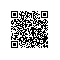# 数据的压缩存储与解压缩算法实现(C语言)

/*
table = tab & 0x0f ;  //取低位
table = tab & 0xf0 ； //取高位
table = tab & 0x0f ;  //取低位
table = tab & 0xf0 ;	//取高位
...
table = tab & 0x0f ;  //取低位
table = tab & 0xf0 ;	//取高位

*/

#include <stdio.h>
unsigned char tab = {0xfe,0xf1,0x3e,0x3c} ;
unsigned char table ;
int main(void)
{
int i ;
int j ;
int count = 0 ;
for(i = 0 ; i < 8 ; i++)
{
if(i % 2 == 0)
table[i] = tab[count] & 0x0f ;
if(i % 2 != 0)
{
table[i] = tab[count] & 0xf0 ;
count++ ;
}
}
for(i = 0 ; i < 8 ; i++)
printf("table[%d]:0x%x\n",i,table[i]) ;
}

运行结果:
table : 0xe
table : 0xf0
table : 0x1
table : 0xf0
table : 0xe
table : 0x30
table : 0xc
table : 0x30 

/*
savebuf = table | table ;
savebuf = table | table ;
savebuf = table | table ;
savebuf = table | table ;
*/

#include <stdio.h>
unsigned char tab = {0xfe,0xf1,0x3e,0x3c} ;
unsigned char table ;
int savebuf ;

int main(void)
{
int i ;
int j ;
int low , high , count = 0 , beat_count ,value ;
count = 0 ;
beat_count = 0 ;
for(j = 0 ; j < 8 ; j++)
{
if(j % 2 == 0)
low = table[j] ;
else
{
high = table[j] ;
count++ ;
savebuf[beat_count] = low | high ;
beat_count++;
}
}
for(i = 0 ; i < 4 ; i++)
printf("savebuf[%d]:%x\n",i , savebuf[i]) ;
} 

运行结果:
savebuf:fe
savebuf:f1
savebuf:3e
savebuf:3c使用钉钉扫一扫加入圈子
+ 订阅